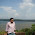## Friday, May 4, 2012

### Analyzing your Gmail with Matplotlib

Lately, I read this post about using Mathematica to analyze a Gmail account. I found it very interesting and I worked a with imaplib and matplotlib to create two of the graph they showed:
• A diurnal plot, which shows the date and time each email was sent (or received), with years running along the x axis and times of day on the y axis.
• And a daily distribution histogram, which represents the distribution of emails sent by time of day.
In order to plot those graphs I created three functions. The first one, retrieve the headers of the emails we want to analyze:
```from imaplib import IMAP4_SSL
from datetime import date,timedelta,datetime
from time import mktime
from email.utils import parsedate
from pylab import plot_date,show,xticks,date2num
from pylab import figure,hist,num2date
from matplotlib.dates import DateFormatter

""" retrieve the headers of the emails
from d days ago until now """
# imap connection
mail = IMAP4_SSL('imap.gmail.com')
mail.select(folder)
# retrieving the uids
interval = (date.today() - timedelta(d)).strftime("%d-%b-%Y")
result, data = mail.uid('search', None,
'(SENTSINCE {date})'.format(date=interval))
result, data = mail.uid('fetch', data.replace(' ',','),
mail.close()
mail.logout()
return data
```
The second one, make us able to make the diurnal plot:
```def diurnalPlot(headers):
""" diurnal plot of the emails,
with years running along the x axis
and times of day on the y axis.
"""
xday = []
ytime = []
if len(h) > 1:
timestamp = mktime(parsedate(h[5:].replace('.',':')))
mailstamp = datetime.fromtimestamp(timestamp)
xday.append(mailstamp)
# Time the email is arrived
# Note that years, month and day are not important here.
y = datetime(2010,10,14,
mailstamp.hour, mailstamp.minute, mailstamp.second)
ytime.append(y)

plot_date(xday,ytime,'.',alpha=.7)
xticks(rotation=30)
return xday,ytime
```
And this is the function for the daily distribution histogram:
```def dailyDistributioPlot(ytime):
""" draw the histogram of the daily distribution """
# converting dates to numbers
numtime = [date2num(t) for t in ytime]
# plotting the histogram
ax = figure().gca()
_, _, patches = hist(numtime, bins=24,alpha=.5)
# adding the labels for the x axis
tks = [num2date(p.get_x()) for p in patches]
xticks(tks,rotation=75)
# formatting the dates on the x axis
ax.xaxis.set_major_formatter(DateFormatter('%H:%M'))
```
Now we got everything we need to make the graphs. Let's try to analyze the outgoing mails of last 5 years:
```print 'Fetching emails...'
'ofcourseiamsupersexy','inbox',365*5)

print 'Plotting some statistics...'
dailyDistributioPlot(ytime)
print len(xday),'Emails analysed.'
show()
```
The result would appear as follows

We can analyze the outgoing mails just using selecting the folder '[Gmail]/Sent Mail':
```print 'Fetching emails...'
'ofcourseiamsupersexy','[Gmail]/Sent Mail',365*5)

print 'Plotting some statistics...'
dailyDistributioPlot(ytime)
print len(xday),'Emails analysed.'
show()
```
And this is the result:

1.Thank you for the article! The concept and the translation to Python is really cool! I will have to try your code out on my own gmail account later.

2.Could you also add how to prevent ipython from storing the email password we typed in the command history?
Loved the script. It is awesome.. :-)

3.Hi Joe, I don't use IPython but you could take a look here:

4.This line not working:

'ofcourseiamsupersexy','[Gmail]/Sent Mail',365*5)

My outbox dir not [Gmail]/Sent Mail.I changed this to outbox solved problem.Thanks

5.This is awesome. Do you know how to access mails stored by thunderbird from python?

1.Sorry Shishir, I don't use Thunderbird.

6.This was really helpful. I'm a newbie with python and I'm not understanding the requirement of this line

'ofcourseiamsupersexy','[Gmail]/Sent Mail',365*5)

Additionally, is it possible to find:

How many emails have I responded within distributed duration like < 1 hr, 1-2 hrs, >2 hrs in specific time frame - Say last 24 hours.

1.Hello wolf, Thanks for you comment. The function get header retrieves the headers of all the emails from d days ago until now.

If you want to retrieve the emails you replied in a certain amount of time you have to get all the email in the inbox and the email in the outbox ('[Gmail]/Sent Mail' in my case) then you can iterate over the received mail and see the closes sent mail to that address. It's not a precise algorithm but it could give you a good approximation.

7.I've got the error. Could you help me with this ?

Traceback (most recent call last):
File "test.py", line 66, in
File "test.py", line 36, in diurnalPlot
timestamp = mktime(parsedate(h[5:].replace('.',':')))
TypeError: argument must be 9-item sequence, not None

1.Hi Youngseok, the function parsedate returns None when it's not able to recognize the string. It may be your case. You should check the input of this function. It may be that the header that you are using is different that the one I used when I wrote this snippet.

8.I keep getting synthax error for

9.